# Finite Volume Simulation of Natural Convection for Power-Law Fluids with Temperature-Dependent Viscosity in a Square Cavity with a Localized Heat Source

Finite Volume Simulation of Natural Convection for Power-Law Fluids with Temperature-Dependent Viscosity in a Square Cavity with a Localized Heat Source

Industrial Engineering Laboratory, Faculty of Sciences and Technologies, Sultan Moulay Slimane University, B.P. 523, Béni-Mellal 23000, Morocco

Laboratory of Applied Mathematics and Computing, Faculty of Sciences and Technologies, Cadi Ayyad University, B.P. 549, Marrakech 40000, Morocco

Team of Applied Physics and New Technologies, Polydisciplinary Faculty, Sultan Moulay Slimane University, B.P. 592, Béni-Mellal 23000, Morocco

Polydisciplinary Faculty, Sultan Moulay Slimane University, B.P. 592, Béni-Mellal 23000, Morocco

Automatic and Energy Conversion and Microelectronics Laboratory, Faculty of Sciences and Technologies, Sultan Moulay Slimane University, B.P. 523, Béni-Mellal 23000, Morocco

Corresponding Author Email:
Page:
1405-1416
|
DOI:
https://doi.org/10.18280/ijht.390502
8 August 2021
|
Accepted:
1 September 2021
|
Published:
31 October 2021
| Citation

OPEN ACCESS

Abstract:

In this paper, numerical study on natural convection heat transfer for confined thermo-dependent power-law fluids is conducted. The geometry of interest is a fluid-filled square enclosure where a uniform flux heating element embedded on its lower wall is cooled from the vertical walls while the remaining parts of the cavity are insulated, without slipping conditions at all the solid boundaries. The governing partial differential equations written in terms of non-dimensional velocities, pressure and temperature formulation with the corresponding boundary conditions are discretized using a finite volume method in a staggered grid system. Coupled equations of conservation are solved through iterative Semi Implicit Method for Pressure Linked Equation (SIMPLE) algorithm. The effects of pertinent parameters, which are Rayleigh number (103 ≤ Ra ≤ 106), power-law index (0.6 ≤ n ≤ 1.4), Pearson number (0 ≤ m ≤ 20) and length of the heat source (0.2 ≤ W ≤ 0.8) on the cooling performance are investigated. The results indicate that the cooling performance of the enclosure is improved with increasing Pearson and Rayleigh numbers as well as with decreasing power-law index and heat source length.

Keywords:

finite volume, natural convection, non-Newtonian fluids, numerical study, square cavity, thermo-dependent viscosity

1. Introduction

Natural convection flows arise from density variations with temperature or concentration within a non-isothermal-fluid under the influence of gravity. This is frequently encountered in many industrial and practical engineering areas that include heat exchangers, nuclear reactors, geothermal systems, metallurgical processes, crystal growth, cooling of electronic components and others.

Among different types of problem configurations, natural convection in closed rectangular or square enclosures has received a special interest of researchers because of its presence in a wide range of applications. One of the characteristics of this heat transfer problem is that, different shapes of flows may take place within the cavity depending on different imposed thermal conditions, which may be presented by either Newmann (imposed heat flux) or Dirichlet (imposed temperature difference) types at the vertical or horizontal walls. Many studies concerning the differentially heated vertical walls case have been investigated for both Newtonian [1, 2] and non-Newtonian fluids [3-11], where the momentum and thermal transports initiate inside the enclosure once a finite temperature difference is occurred between the side walls. When the lower wall of the enclosure is maintained at a higher temperature than the top wall while the sidewalls are adiabatic, the classical Rayleigh-Bénard convection is observed within the cavity which starts only when a critical Rayleigh number is exceeded. The classical Rayleigh-Bénard convection has also been studied for both Newtonian  and different types of non-Newtonian fluids such as viscoelastic , power law [14-16] and viscoelastic [17-19] fluids.

Additionally to the classical Rayleigh-Bénard convection problem when the entire bottom wall is heated, considerable numerical and experimental studies have been performed for natural convection of confined Newtonian fluids in enclosures with a partially heated bottom wall. The problem is frequently encountered in many engineering applications such as cooling of electronic equipment and others, where the discrete heat source can simulate chips . It is evident that the heating element length controls the total heat transferred inside the enclosure and this is strongly dependent on the nature of thermal conditions of the heat source. In general, the configurations with discrete heat source are divided into two main categories depending on the type of thermal conditions of heat source, heat source producing a constant temperature or a uniform heat flux where the temperature is not constant at the heated part. For the case where the heating element provides a constant temperature, Aydin and Yang  have numerically investigated natural convection of air confined in a square cavity with localized heating from below, which is considered to be isothermally heated at a constant temperature, and symmetrical cooling from the vertical walls, while the upper one and the rest of the bottom wall are adiabatic. Finite difference procedure has been used to solve the governing equations. It has been found that an increase in Rayleigh number and heat source length enhances the heat transfer inside the enclosure. Thereafter, further studies have been conducted to analyze different effects such as heat source position, aspect ratio, inclination of the enclosure and others may be found in [21-29]. For the second category when the heat source produces a uniform heat flux, Sharif and Mohammad  have numerically studied the same configuration as , where the heat source at the lower wall has been replaced with a uniform heat flux source. They have also analyzed the effects of inclination and aspect ratio of the enclosure. It has been noticed that the heat transfer decreases with increasing heat source length, as well as the inclination of the cavity has a significant effect only for higher aspect ratios. Additional relevant papers, which have also treated natural convection with localized uniform heat flux source at the bottom wall, considering different thermal conditions and supplemental effects for Newtonian fluids may be found in [31-34].

Cooling heat source problem has also been dealt with non-Newtonian fluids because many situations require cooling efficiency improvement, and thus an amelioration of heat transfer is needed, in order to maintain the adequate working conditions and not to surpass certain permitted temperatures. For the heat source producing a constant temperature, Hassan et al.  have numerically investigated natural convection problem in a square cavity whose vertical walls are maintained at a cold temperature and the top wall is adiabatic, while a heating element is located at the lower wall. The cavity is filled with viscoplastic fluids modeled by Bingham fluids. It has been found that the increase in Bingham number causes the convection effect and the fluid circulation to decrease inside the cavity due to the augmentation of flow resistance. This last configuration has also been studied by Yigit et al.  by using power-law fluids. It has been noticed that the enclosure performs better with shear-thinning fluids (n < 1) from the perspective of enhancing heat transfer performance. For the heat source providing a uniform heat flux, Aminosadati and Ghasemi  have numerically analyzed natural convection for nanofluids confined in a square enclosure with localized heat source embedded on the bottom wall, producing a uniform heat flux, while the top and vertical walls are maintained at a relatively low temperature. They have investigated the effects of the type of nanofluid and solid volume fraction of nanoparticles. It has been found that adding nanoparticles into the base fluid causes the Nusselt number to increase and the heat source maximum temperature to decrease, which means an improvement in the cooling power of the enclosure. Raisi  has numerically studied the same configuration treated in  where the nanofluid has been replaced with power-law fluids. The effects of power-law index and Rayleigh number have been examined. It has been found that the decrease in power-law index and increase in Rayleigh number improve the cooling efficiency through the increase and decrease in the average Nusselt number and heat element maximum temperature, respectively. Recently, Hassan et al.  have computationally and experimentally studied the problem treated in  by using Bingham fluids. It has been noticed that the convective transport drops gradually with the increase in fluid yield stress, as well as the convection process is stronger in the case of constant temperature lower wall heating compared to the uniform heat flux bottom wall heating case.

Majority of the published studies dealing with natural convection cooling of a localized heat source are customarily based on the common assumption that the fluid properties are constant with respect to the temperature except for the fluid density in the buoyancy force term, which obeys the Boussinesq approximation. However, for many real fluids, the viscosity is effectively dependent upon temperature. Natural convection inside horizontally heated enclosures, considering thermo-dependent physical parameters, has been studied for both Newtonian [40, 41] and non-Newtonian [42-45] fluids. It has been noticed that an increase in the thermo-dependency parameter leads to an augmentation of the convection current and heat transfer inside the cavity. However, only few investigations on Buoyancy-driven convection for thermo-dependent non-Newtonian fluids confined in enclosures heated from bellow have been performed. Abu-Nada  has analyzed Rayleigh-Bénard convection for nanofluids with temperature-dependent physical parameters, which are the viscosity and thermal conductivity. The problem has been numerically studied using a finite volume technique. Kaddiri et al.  have numerically investigated Rayleigh-Bénard convection of power-law fluids with temperature-dependent viscosity. The governing equations have been numerically solved using a finite difference approach. It has been reported that the flow loses its centro-symmetry due to the temperature-dependent parameter, and the streamline core moves toward the region where the effective viscosity is lower. It has also been noted that the thermo-dependency parameter tends to make precocious the convection because of the drop in the value of critical Rayleigh number with increasing Pearson number.

From the above mentioned literature survey, it has been noticed that, there is no study on natural convection cooling of a localized heat source placed on the lower wall of an enclosure containing thermo-dependent non-Newtonian power-law fluids. Since the viscosity of thermo-dependent fluids decreases with increasing thermo-dependency parameter, the enclosure is expected to perform better with these fluids from the perspective of heat transfer efficiency enhancement. Consequently, this lack has been treated in the present study by numerical simulations for natural convection of power-law fluids with thermo-dependent viscosity in a square enclosure with a partial localized heating, which is achieved by a heat source producing a uniform heat flux and placed at the center of the bottom wall, and symmetrical cooling from the sidewalls. Thus, the main purpose of the present work is to examine the effects of several pertinent parameters such as power-law index, Rayleigh number, Pearson number and heat source length on the fluid flow and the resulting heat transfer.

2. Problem Description

The physical model under consideration is depicted in Figure 1. It consists of a two-dimensional square cavity of size L x L, whose vertical walls are maintained at a cold temperature $T_{c}^{\prime}$. A heat source of variable length l producing a constant heat flux is located in the center of the bottom wall, while the remaining parts of the lower wall and the top wall are adiabatic, without slipping conditions at all the solid boundaries. The cavity contains non-Newtonian fluid, whose rheological behavior can be expressed by the power-law model, proposed by Ostwald-de Waele, which, in terms of viscous stress tensor, can be presented as:

## 1.png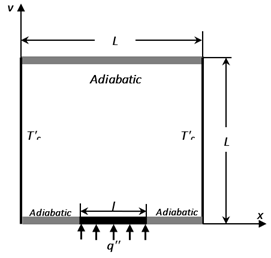Figure 1. Sketch of the cavity and boundary conditions

${{\tau }_{ij}}=2{{\mu }_{{{a}'}}}{{D}_{ij}}={{\mu }_{{{a}'}}}\left( \frac{\partial {{{{U}'}}_{i}}}{\partial {{x}_{j}}}+\frac{\partial {{{{U}'}}_{j}}}{\partial {{x}_{i}}} \right)$      (1)

Here Dij stands for the rate of strain tensor for the two-dimensional Cartesian coordinate and $\mu_{a}^{\prime}$ is the apparent viscosity, which is defined as:

${{\mu }_{{{a}'}}}={{K}_{_{T}}}{{\left\{ 2\left[ {{\left( \frac{\partial {U}'}{\partial x} \right)}^{2}}+{{\left( \frac{\partial {V}'}{\partial y} \right)}^{2}} \right]+{{\left( \frac{\partial {V}'}{\partial x}+\frac{\partial {U}'}{\partial y} \right)}^{2}} \right\}}^{\frac{n-1}{2}}}$      (2)

where the two empirical parameters, n and KT, denote the flow behavior and consistency indices, respectively. In general, they are functions of the temperature, but often the temperature dependence of n is very weak compared to that of KT , which can be expressed by the following exponential law :

${{K}_{_{T}}}=k\,\,\exp \,\left( -b({T}'-{{{{T}'}}_{r}}) \right)$      (3)

where, b is the thermo-dependency parameter and k is the consistency index at the reference temperature $T_{r}^{\prime}$. Moreover, for n < 1 the apparent viscosity decreases with increasing the shear rate and thus the behavior is shear-thinning. Inversely, for n > 1 the apparent viscosity increases with increasing the shear rate and the fluid is referred to as shear-thickening. For n = 1 a Newtonian fluid is obtained and the consistency is just the viscosity.

3. Governing Equations

It is considered that the cavity is filled with thermo-dependent power law fluids, the flow is assumed to be laminar and steady, the fluid density obeys the Boussinesq approximation, and the viscous dissipation effects are negligible.

Under the aforementioned assumptions, and by using the following characteristic scales, in which $U^{\prime}, V^{\prime}$ are the velocity components in $x, y$-direction, $T^{\prime}$ represents the dimensional temperature, and $\alpha$ is the thermal diffusivity:

$X=\frac{x}{L},\ Y=\frac{y}{L},\ U=\frac{{U}'\,L}{\alpha },\ V=\frac{{V}'\,L}{\alpha },\ T=\frac{{T}'-{{{{T}'}}_{c}}}{\Delta T*}\ \ and\ \Delta T*=\frac{{{q}''}}{\lambda L}$

The dimensionless governing equations written in terms of velocity vector components, (U, V), pressure, P, and temperature, T, in Cartesian coordinate system (X, Y), are:

$\frac{\partial U}{\partial X}+\frac{\partial V}{\partial Y}=0$      (4)

$U \frac{\partial U}{\partial X}+V \frac{\partial U}{\partial Y}=-\frac{\partial P}{\partial X}+\operatorname{Pr}\left[\mu_{a}\left(\frac{\partial^{2} U}{\partial X^{2}}+\frac{\partial^{2} U}{\partial Y^{2}}\right)+2 \frac{\partial \mu_{a}}{\partial X} \frac{\partial U}{\partial X}+\frac{\partial \mu_{a}}{\partial Y}\left(\frac{\partial U}{\partial Y}+\frac{\partial V}{\partial X}\right)\right]$      (5)

$U \frac{\partial V}{\partial X}+V \frac{\partial V}{\partial Y}=-\frac{\partial P}{\partial Y}$$+\operatorname{Pr}\left[\begin{array}{l}R a T+\mu_{a}\left(\frac{\partial^{2} V}{\partial X^{2}}+\frac{\partial^{2} V}{\partial Y^{2}}\right) \\ +2 \frac{\partial \mu_{a}}{\partial Y} \frac{\partial V}{\partial Y}+\frac{\partial \mu_{a}}{\partial X}\left(\frac{\partial U}{\partial Y}+\frac{\partial V}{\partial X}\right)\end{array}\right]$      (6)

$U\frac{\partial T}{\partial X}+V\frac{\partial T}{\partial Y}=\frac{{{\partial }^{2}}T}{\partial {{X}^{2}}}+\frac{{{\partial }^{2}}T}{\partial {{Y}^{2}}}$      (7)

where:

{{\mu }_{a}}=\exp \,\,\left( -mT \right)\,\,{{\left\{ \begin{align} & 2\,\,\left[ {{\left( \frac{\partial U}{\partial X} \right)}^{2}}+{{\left( \frac{\partial V}{\partial Y} \right)}^{2}} \right] \\ & +{{\left( \frac{\partial U}{\partial Y}+\frac{\partial V}{\partial X} \right)}^{2}} \\\end{align} \right\}}^{\frac{n\,\,-1}{2}}}      (8)

The corresponding boundary conditions are:

$U=V=T=0\quad for\quad X=0\ and\ 0\le Y\le 1$      (9)

$U=V=T=0\quad for\quad X=1\ and\ 0\le Y\le 1$     (10)

$U=V=\frac{\partial T}{\partial Y}=0\quad for\quad Y=1\ and\ 0\le X\le 1$     (11)

\begin{align} & U=V=\frac{\partial T}{\partial Y}+1=0\text{ }for\text{ }Y=0\ and \\ & 0.5-\frac{W}{2}\le X\le 0.5+\frac{W}{2} \\\end{align}      (12)

U=V=\frac{\partial T}{\partial Y}=0\quad for\quad Y=0\ and\ \left\{ \begin{align} & 0\,<\,X\,<\,0.5-\frac{W}{2} \\ & 0.5+\frac{W}{2}\,<\,X\,<\,1 \\\end{align} \right.       (13)

The dimensionless parameters appearing now in the present problem are:

$\Pr =\frac{\left( {k}/{\rho }\; \right)\,{{L}^{2-2n}}}{{{\alpha }^{2-n}}}\quad \quad \left( Prandtl number \right)$      (14)

$Ra=\frac{g\beta {{L}^{2-2n}}q''}{\left( {k}/{\rho }\; \right)\,\lambda {{\alpha }^{n}}}\quad \quad \left( Rayleigh number \right)$      (15)

\begin{align} & m=b\Delta T*\,=\ -\frac{1}{{{K}_{T}}}\frac{d{{K}_{T}}}{T} \\ & =\frac{d\left( \,\ln \,\left( {{{K}_{T}}}/{k}\; \right) \right)}{dT}\ \,\left( Pearson number \right) \\\end{align}      (16)

$W=\frac{l}{L}\quad \quad \left( heater dimensionless length \right)$        (17)

The steady solution has been used to measure the heat transfer rate of the cavity by calculating the local Nusselt number on the heat source element defined as follow:

$Nu=\frac{hL}{k}=\frac{q''L}{\left( {{{{T}'}}_{_{S}}}(x)-{{{{T}'}}_{c}} \right)\,\,k}$       (18)

where, h is the convection heat transfer coefficient. By using the dimensionless parameters, the Eq. (18) becomes:

$Nu=\frac{1}{{{T}_{_{S}}}(X)}$      (19)

Here, Ts(X) is the dimensionless temperature of heating element. The average Nusselt is obtained by integrating Eq. (19) along the heat source.

$\overline{Nu}=\frac{1}{W}\int\limits_{0.5-\frac{W}{2}}^{0.5+\frac{W}{2}}{Nu\ dX}$      (20)

4. Numerical Method

All of the governing differential Eqns. (4)-(7) can be written in the following form of the general transport Eq. :

$\frac{\partial }{\partial X}\left( U\Phi -\Gamma \frac{\partial \Phi }{\partial X} \right)+\frac{\partial }{\partial Y}\left( V\Phi -\Gamma \frac{\partial \Phi }{\partial Y} \right)={{S}_{\Phi }}$      (21)

where, Φ is the working variable which represents U, V or $\Gamma$ is $\operatorname{Pr} \mu_{a}$ for the momentum equations and 1 for the energy equation, and SΦ is the source term which includes all the terms that cannot be accommodated in the convective and diffusion terms.

The Eq. (21) has to be converted into a linear algebraic equation in order to obtain a numerical solution. Consequently, a finite volume approach is considered for the spatial discretization . The finite volume method can be advantageous in terms of computer space and time requirements as well as in terms of numerical stability. For this method, the computational field is divided into a set of control volumes ΔV = ΔX x ΔY around nodes P (Figure 2), where ΔX and ΔY are the length-step of both X and Y directions.

By integrating Eq. (21) over a control volume and using the divergence theorem , the final form of the discretized equations relating the variable Φp to its neighboring grid point values can be written in each control volume as:

${{A}_{P}}{{\Phi }_{P}}={{A}_{W}}{{\Phi }_{W}}+{{A}_{E}}{{\Phi }_{E}}+{{A}_{S}}{{\Phi }_{S}}+{{A}_{N}}{{\Phi }_{N}}+{{S}_{\varphi }}$      (22)

Table 1. Average Nusselt number at the heat source and maximum stream function inside the enclosure for different meshes

 Number of grids $\overline{Nu}$ ${{\Delta }_{_{1}}}=\frac{\left| {{\overline{Nu}}_{_{i\,\times j}}}\text{ }-{{\overline{Nu}}_{_{150\,\times \,150\quad}}}\text{ } \right|}{{{\overline{Nu}}_{_{150\,\times \,150}}}}\text{ }\times 100\ %$​ Ψmax ${{\Delta }_{_{2}}}=\frac{\left| {{\left. {{\Psi }_{\max }}\text{ } \right)}_{_{i\,\times \,j}}}\text{ }-{{\left. {{\Psi }_{\max }}\text{ } \right)}_{_{150\,\times 150\quad}}}\text{ } \right|}{{{\left. {{\Psi }_{\max }}\text{ } \right)}_{_{150\,\times \,150}}}}\text{ }\times 100\ %$ 150 x 150 26.6764 --- 37.6630 --- 200 x 200 26.6298 0.17 37.6873 0.06 250 x 250 26.6058 0.26 37.6878 0.06 300 x 300 26.5905 0.32 37.6754 0.03

Table 2. Comparison of our simulation results with previous studies for different values of Gr and W

 Grashof number 103 104 105 106 W=0.2 $\overline{N u}$ Present study 5.9936 6.0037 7.2051 11.4249 Sharif et al.  5.9266 5.9463 7.1241 11.3415 Error (%) 1.1 0.9 1.1 0.7 Tmax Present study 0.1818 0.1819 0.1564 0.1086 Sharif et al.  0.1819 0.1818 0.1568 0.1092 Error (%) 0.05 0.05 0.2 0.5 W=0.8 $\overline{N u}$ Present study 3.5934 3.7276 5.9055 9.2785 Sharif et al.  3.5562 3.6919 5.8644 9.2880 Error (%) 1 0.9 0.7 0.1 Tmax Present study 0.3612 0.3653 0.2628 0.1775 Sharif et al.  0.3637 0.3674 0.2651 0.1792 Error (%) 0.6 0.5 0.8 0.9

## 2.png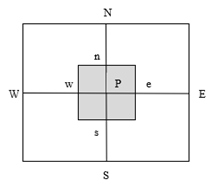Figure 2. Control volume

4.1 Solution method

The obtained discretized equations for every control volume in the computational field consist of a set of linear algebraic equations, which then can be solved iteratively by means of the line by line technique based on the tridiagonal matrix algorithm (TDMA) [50, 51].

Since the pressure is an unknown in the momentum equations, a derivation of pressure equation is evidently required to solve the discretized equations for obtaining the temperature and kinematic fields. Consequently, Semi-Implicite method for pressure linked equation (SIMPLE) algorithm , which allows the transformation of the continuity equation to the pressure equation, is used. In order to avoid physically unrealistic solutions, such as the checkerboard pressure and velocity distribution, the staggered grid system is imposed.

The solution process is reiterated until attaining the convergence criterion:

$\operatorname{Max}\left(\frac{\Phi^{n+1}-\Phi^{n}}{\Phi^{n+1}}\right) \leq 10^{-7}$     (23)

where, Φ = (U, V, T).

4.2 Mesh independency

To obtain a mesh independent solution, a mesh sensitivity study is conducted for the natural convection in the enclosure shown in Figure 1. Table 1 exhibits the effects of grid points number on $\overline{Nu}$ and Tmax at the heated surface for four different mesh combinations at the following control parameters: Ra = 106, n = 0.6, m = 20 and W = 0.4. A uniform grid of 150 x 150 is found to present sufficient precision and reasonable computational time.

4.3 Validation of the numerical code

To validate the elaborated numerical code, our results, expressed in terms of $\overline{N u}$ (average Nusselt number) and Tmax (heat source maximum temperature), are compared with previous researches for both Newtonian and power-law fluids. Published studies for similar configuration and boundary conditions undertaken in the present work are only available for Newtonian fluids. Thus, direct validation of the present simulation against the results of Sharif et al.  was performed for Newtonian fluid at various Grashof numbers, defined as Gr = Ra / Pr, and two different lengths of the heat source. For non-Newtonian fluids, the present numerical code was also validated against the investigation of Raisi , where the top and vertical walls are maintained at a relatively low temperature, at W = 0.4 and different values of n and Ra. Hence, as can be seen from Table 2 and Figure 3 a good agreement is generally obtained.

## 3.png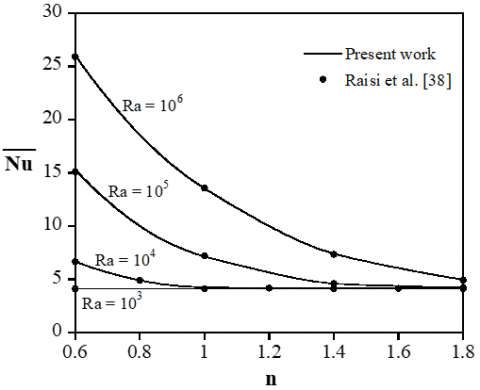Figure 3. Comparison of our simulation results with those of Raisi  at Pr = 100 and different values of Ra and n

5. Results and Discussion

Numerical simulations have been conducted for the control parameters defined earlier, namely, The Rayleigh number, Ra, the power-law index, n, the Pearson number, m, and the heat source length, W, that vary in the following ranges: 103 ≤ Ra ≤ 106, 0.6 ≤ n ≤ 1.4, 0 ≤ m ≤ 20 and 0.2 ≤ W ≤ 0.8, respectively. The effect of the Prandtl number is still ignored since the Pr number for the non-Newtonian fluids considered in the present work is sufficiently large, and a rise of this parameter in such condition makes the influence of the inertia terms negligible and the heat transfer and fluid flow unchanged, as has been demonstrated by Lamsaadi et al. , Yigit et al.  and many others. Thus, the Pr number was kept constant and equal 100 for the rest of this study.

5.1 Effects of power-law index, Rayleigh and Pearson numbers

In this section of the study, the effects of the power law index, n, the Rayleigh number, Ra, and the Pearson number, m, are examined on the fluid flow and the resulting heat transfer. However, the heat source length was kept at a constant value W = 0.4.

Figures 4 and 5 present the streamlines and isotherms respectively for multifarious power law indexes, Rayleigh and Pearson numbers. Since the heat source is placed in the middle of the lower wall, two symmetrical counter-rotating cells are created inside the enclosure regardless of the considered control parameters. It is clear from Figure 4 that the increase in Rayleigh number causes the magnitude of Ψ to decrease because of the strengthening of buoyancy forces in comparison to viscous forces. Moreover, Figure 4 also illustrates that the decrease in n gives rise to the weakening of viscous flow resistance in comparison to convective transport, which is reflected in the increase in the circulating cells intensity for smaller power law indexes. Therefore, the greatest flow intensity occurs at the lowest and highest values of n and Ra, respectively. However, a poor convection is formed inside the enclosure at Ra = 103. As a consequence, the flow does not undergo significant qualitative and quantitative changes when n is varied in its range due to the fact that the buoyancy forces are not yet sufficient to promote the flow intensity indicating that the pseudo-conductive regime is still dominant. Nevertheless, the effect of n is well visible with increasing Ra. Furthermore, Figure 4 also exhibits the explicit impact of the temperature-dependent viscosity on the flow structure. Figure 4 shows that the strength of the rotating cells increases with the enhancement of Pearson number due to the weakening of apparent viscosity as m increases. In addition, the convective cells cores move closer to the middle of the heat source -in which the temperature takes its maximum value inside the enclosure (see Sharif et al. )- as a consequence of the apparent viscosity decrease in this region. At the same time, the effect of Pearson number becomes less pronounced for high and low values of Ra and n, respectively, indicating that the influence of m is strongly dependent on transport regime.

## 4.png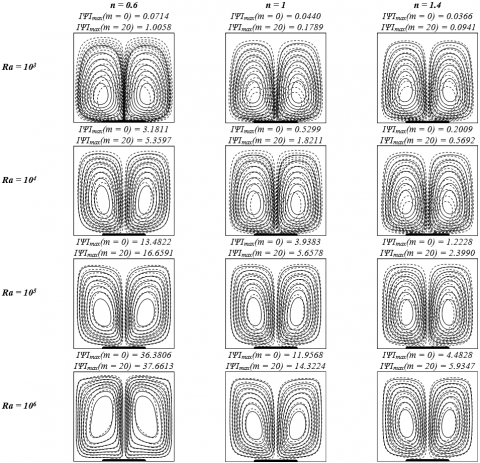Figure 4. Streamlines for different values of Rayleigh number, power law index, m = 0 (━━) and m = 20 (- - -)

According to Figure 5, the isotherms also have symmetrical forms for all cases. At Ra = 103, a weak convection is created inside the enclosure as indicated by parabolic isotherms. By increasing Rayleigh number and decreasing n, the thermal and dynamic forces are enhanced, which clearly modifies the shape of isotherms and gives rise to the creation of a thermal plume. Consequently, the heat transfer within the enclosure is improved, thereby decreasing the temperature of the heat source and inside the cavity. Besides, it is clear that the increase in m alters the form of the isotherms as they traverse more distance, and an augmentation of m leads to a distortion of isotherms toward the strong convection region where the apparent viscosity is smaller. Moreover, as the m parameter is increased, the maximum temperature inside the enclosure is decreased. Therefore, this indicates the enhancement of heat transfer by the Pearson number augmentation.

Figure 6 shows the dimensionless vertical velocity along the enclosure’s mid-section at Ra = 105. It is observed symmetrical velocity profiles indicating the direction of the flow inside the cavity. The flow rises in the middle and descends downwards near the sidewalls. It is also clear that an increase in m and a decrease in n result in the enhancement of the vertical velocity intensity as a result of the decrease in the apparent viscosity. Moreover, for the highest value of n and m = 0, the velocity tends to be feeble due to the high apparent viscosity.

## 5.pngFigure 5. Isotherms for different values of Rayleigh number, power law index, m = 0 (━━) and m = 20 (- - -)

## 6.png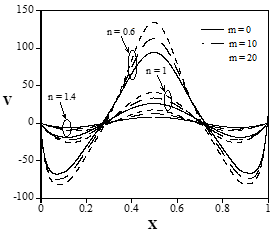Figure 6. Vertical velocity profiles along the mid-length of the cavity for various values of power-law index, Pearson number and Ra = 105

The temperature of the heating element with a constant heat flux, such as electronic components, is not uniform along this element and contains a maximum value. This maximum temperature of electronic components is a critical factor since it may be detrimental to their life and performance. It is therefore useful to investigate the heat source maximum temperature closely. Figure 7(a) displays the maximum temperature inside the enclosure, for different power-law indexes, Rayleigh and Pearson numbers. Since a poor convection is formed inside the enclosure at Ra = 103, the Pearson number and the power-law index have only a negligible effect on the heat source maximum temperature. However, at higher Rayleigh numbers, a decreased n and increased m lead to the strengthening of convection process inside the enclosure, and thus, the fluid takes more heat away from the heating element which results in decreasing the heat source maximum temperature. These statements are also supported by Figure 7(b) which shows that the average Nusselt number is not affected by the variations of power-law index and Pearson number when conduction is the dominant mode of heat transfer (Ra=103), whereas, at higher Rayleigh numbers, $\overline{N u}$ increases with increasing m and decreasing n due to the weakening of the fluid apparent viscosity, and thus, the effects of natural convection become stronger. According to Figures 7(a) and (b), the effect of thermo-dependency parameter on the heat source maximum temperature and the average Nusselt number become less pronounced at the highest and smallest values of Ra and n, respectively, which is quite obvious since strong convection inhibits such an effect.

## 7.png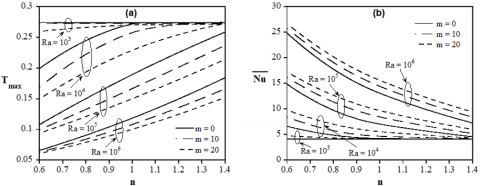Figure 7. Variations of heat source maximum temperature Tmax (a) and average Nusselt number $\overline{N u}$  (b) for different values of Rayleigh number, power-law index and Pearson number

## 8.png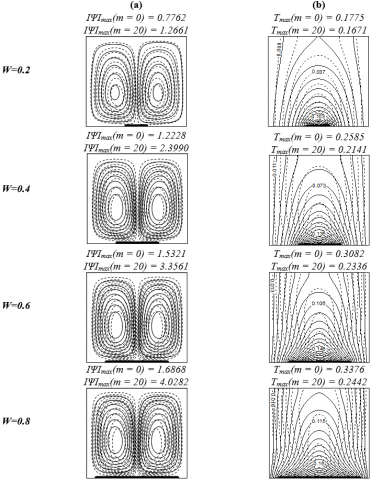Figure 8. Streamlines (a) and Isotherms (b) for various heat source lengths, m = 0 (━━) and m = 20 (- - -) (Ra = 105 and n = 1.4)

5.2 Effects of heat source length

In this section, the effects of the lengths of heat source placed in the center of the lower wall, on the fluid flow and the cooling efficiency inside the enclosure are evaluated for different values of the fluid parameters, where Ra was kept at a constant value Ra = 105.

## 9.png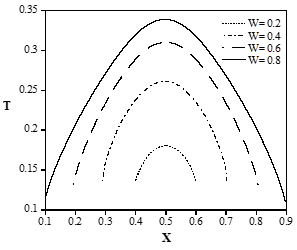Figure 9. Variations of heat source temperature for different values of heat source length. (m = 0, n = 1.4 and Ra = 105)

Figure 8 presents the effects of heat source lengths on the streamlines (a) and corresponding isotherms (b) at Ra = 105n = 1.4 and various Pearson numbers. It can be seen from Figure 8 that an increase in the heat source length leads to an intensification of the rotating cells intensity because of the rise of the heat generation as the length of heat source increases. Moreover, at W = 0.2 and m = 0, parabolic isotherms are formed within the cavity indicating that the heat transfer principally takes place by conduction. The isotherms start to be more curved with increasing W as a result of the strengthening of convective transport in spite of the increase in the maximum temperature inside the enclosure. Furthermore, a visual examination of isotherms shows that the effect of Pearson number is phenomenally more visible for higher lengths, and also, at W = 0.8, a growth of m from 0 up to 20 leads to an augmentation of ІΨІmax and diminution of Tmax at 138% and 38%, respectively, this variation is more important compared to the case when W = 0.2, where a raise of m from 0 to 20 results in the rise of ІΨІmax at 63% while Tmax reduces at 6.2%.

It is useful to inspect the non-dimensional temperature of the heat source in order to have a better understanding of the influence of heat source lengths on the heat transfer rate. The distribution of dimensionless temperature T along the heating element at n = 1.4 and m = 0 is shown in Figure 9 for various values of W ranging from 0.2 to 0.8. It is apparent from Figure 9 that the heat source temperature increases with the rise of the length of heating element due to higher total heat transferred as the heat source length increases. This trend confirms that the rise of heat source length reduces the cavity cooling performance despite the enhanced convective transport (Figure 8).

Figure 10 exhibits the effects of heat source lengths, power law index and Pearson number on the heat source maximum temperature and average Nusselt number. It is evident that the decrease in n leads to an enhancement of heat transfer inside the enclosure which is reflected in the reduction of the maximum temperature of the heat source and augmentation of the average Nusselt number. Figure 10 also shows that the maximum temperature increases with increasing heat source length, which is evident since the heat source temperature is upgraded as the heat source length increases (Figure 9) due to the greater heat generation rates. Consequently, the average Nusselt number decreases slightly with the increase in the length of the heat source in spite of the enhanced convection. Moreover, the increase in m enhances the cooling power of the fluid, which is indicated by the decrease in the maximum temperature inside the enclosure as a result of the decrease in the viscous forces. Furthermore, the effect of m is more pronounced for higher heat source lengths, which is evident since the thermo-dependency impact manifests itself when the temperatures inside the enclosure are more important and the conduction regime is surpassed.

## 10.png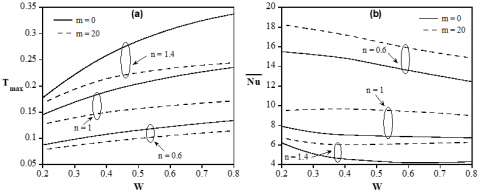Figure 10. Variations of heat source maximum temperature Tmax (a) and average Nusselt number $\overline{N u}$  (b) for different values of heat source length, power-law index and Pearson number. (Ra = 105)

6. Conclusion

In the current investigation, a numerical study has been performed to investigate the steady state, natural convection for temperature-dependent power-law fluids in a square enclosure partially heated from the lower wall and symmetrically cooled from the vertical sides. The impacts of different control parameters, which are Rayleigh number, Power-law index, Pearson number and heated source length, on heat transfer performance and fluid flow inside the cavity have been analyzed. It has been noticed that the average Nusselt number increases and the heat source maximum temperature decreases with increasing Rayleigh number due to the strengthening of convective transport. Decreasing the power-law index and increasing the Pearson number reduces the apparent viscosity of the fluid, which leads to the enhancement of convection transport due to weakening of resistance to the fluid motion offered by the fluid viscosity. Moreover, the effects of m and n are more pronounced when the conduction-regime is exceeded and a strong convection inhibits the effect of m. Furthermore, increasing the heat source length enhances the convective transport inside the enclosure, but simultaneously increases the maximum temperature of the heat source. The present results have also demonstrated that the usage of Newtonian or non-Newtonian fluids with high Pearson number can be an efficient option to enhance the cooling performance for the configuration considered in the present work.

Acknowledgment

The current study was supported through computational resources of HPC-MARWAN (High Performance Computing-Moroccan Academic and Research Wide Area Network) provided by CNRST (the National Center for Scientific and Technical Research), Rabat, Morocco.

Nomenclature
 b thermo-dependency coefficient, Eq. (3) g gravitational acceleration (m/s2) k consistency index for a power-law fluid, Eq. (3) l heat source length (m) L height or width of the enclosure (m) m Pearson number, Eq. (16) n power law index, Eq. (2) Nu local Nusselt number, Eq. (18) and (19) $\overline{N u}$ mean Nusselt number, Eq. (20) P dimensionless pressure Pr generalized Prandtl number, Eq. (14) q'' constant heat flux (W.m-2) Ra generalized Rayleigh number, Eq. (15) T dimensionless temperature T' dimensional temperature (K) W dimensionless heat source length, Eq. (17) (U,V) dimensionless horizontal and vertical velocities (U',V') horizontal and vertical velocities (m/s) (X,Y) dimensionless horizontal and vertical coordinates (X',Y') horizontal and vertical coordinates (m) Greek symbols $\alpha$ thermal diffusivity (m2/s) $\beta$ thermal expansion coefficient (K-1) $\mu_{a}^{\prime}$ apparent viscosity of fluid, Eq. (2) (kg/(m.s)) $\mu_{a}$ dimensionless apparent viscosity of fluid, Eq. (8) $\lambda$ thermal conductivity (W/(m.K)) $\rho$​ density of fluid (kg/m3) $\Psi$ dimensionless stream function $\Gamma$ diffusion coefficient, Eq. (21) $\phi$ working variable, Eq. (21) Subscripts a apparent variable max maximum value Superscripts ' dimensional variables
References

 De Vahl Davis, G. (1983). Natural convection of air in a square cavity: A bench mark numerical solution. International Journal for Numerical Methods in Fluids, 3(3): 249-264. https://doi.org/10.1002/fld.1650030305

 Corvaro, F., Paroncini, M., Sotte, M. (2012). PIV and numerical analysis of natural convection in tilted enclosures filled with air and with opposite active walls. International Journal of Heat and Mass Transfer, 55(23-24): 6349-6362. https://doi.org/10.1016/j.ijheatmasstransfer.2012.06.003

 Lamsaadi, M., Naimi, M., Hasnaoui, M., Mamou, M. (2006). Natural convection in a vertical rectangular cavity filled with a non-Newtonian power law fluid and subjected to a horizontal temperature gradient. Numerical Heat Transfer, Part A: Applications, 49(10): 969-990. https://doi.org/10.1080/10407780500324988

 Lamsaadi, M., Naimi, M., Hasnaoui, M. (2006). Natural convection heat transfer in shallow horizontal rectangular enclosures uniformly heated from the side and filled with non-Newtonian power law fluids. Energy Conversion and Management, 47(15-16): 2535-2551. https://doi.org/10.1016/j.enconman.2005.10.028

 Lamsaadi, M., Naimi, M., Hasnaoui, M., Mamou, M. (2006). Natural convection in a tilted rectangular slot containing Non-Newtonian Power-Law fluids and subject to a longitudinal thermal gradient. Numerical Heat Transfer, Part A: Applications, 50(6): 561-583. https://doi.org/10.1080/10407780600599513

 Turan, O., Chakraborty, N., Poole, R.J. (2010). Laminar natural convection of Bingham fluids in a square enclosure with differentially heated side walls. Journal of Non-Newtonian Fluid Mechanics, 165(15-16): 901-913. https://doi.org/10.1016/j.jnnfm.2010.04.013

 Turan, O., Sachdeva, A., Chakraborty, N., Poole, R.J. (2011). Laminar natural convection of power-law fluids in a square enclosure with differentially heated side walls subjected to constant temperatures. Journal of Non-Newtonian Fluid Mechanics, 166(17-18): 1049-1063. https://doi.org/10.1016/j.jnnfm.2011.06.003

 Turan, O., Sachdeva, A., Poole, R.J., Chakraborty, N. (2012). Laminar natural convection of power-law fluids in a square enclosure with differentially heated sidewalls subjected to constant wall heat flux. Journal of Heat Transfer, 134(12): 122504. https://doi.org/10.1115/1.4007123

 Sheremet, M.A., Pop, I. (2018). Natural convection combined with thermal radiation in a square cavity filled with a viscoelastic fluid. International Journal of Numerical Methods for Heat & Fluid Flow, 28(3): 624-640. https://doi.org/10.1108/HFF-02-2017-0059

 Devi, T.S., Lakshmi, C.V., Venkatadri, K., Prasad, V.R., Bég, O.A., Reddy, M.S. (2020). Simulation of unsteady natural convection flow of a Casson viscoplastic fluid in a square enclosure utilizing a MAC algorithm. Heat Transfer, 49(4): 1769-1787. https://doi.org/10.1002/htj.21690

 Daghab, H., Kaddiri, M., Raghay, S., Lamsaadi, M., El Harfi, H. (2021). Numerical study of natural convection for generalized second-grade fluids confined in a square cavity subjected to horizontal heat flux. International Journal of Heat and Technology, 39(2): 345-354. https://doi.org/10.18280/ijht.390203

 Ouertatani, N., Cheikh, N.B., Beya, B.B., Lili, T. (2008). Numerical simulation of two-dimensional Rayleigh–Bénard convection in an enclosure. Comptes Rendus Mécanique, 336(5): 464-470. https://doi.org/10.1016/j.crme.2008.02.004

 Demir, H. (2003). Rayleigh–Benard convection of viscoelastic fluid. Applied Mathematics and Computation, 136(2-3): 251-267. https://doi.org/10.1016/S0096-3003(02)00036-X

 Lamsaadi, M., Naimi, M., Hasnaoui, M. (2005). Natural convection of non-Newtonian power law fluids in a shallow horizontal rectangular cavity uniformly heated from below. Heat and Mass Transfer, 41(3): 239-249. https://doi.org/10.1007/s00231-004-0530-8

 Turan, O., Lai, J., Poole, R.J., Chakraborty, N. (2013). Laminar natural convection of power-law fluids in a square enclosure submitted from below to a uniform heat flux density. Journal of Non-Newtonian Fluid Mechanics, 199: 80-95. https://doi.org/10.1016/j.jnnfm.2013.06.002

 Yigit, S., Poole, R.J., Chakraborty, N. (2015). Effects of aspect ratio on laminar Rayleigh–Bénard convection of power-law fluids in rectangular enclosures: A numerical investigation. International Journal of Heat and Mass Transfer, 91: 1292-1307. https://doi.org/10.1016/j.ijheatmasstransfer.2015.08.032

 Turan, O., Chakraborty, N., Poole, R.J. (2012). Laminar Rayleigh-Bénard convection of yield stress fluids in a square enclosure. Journal of Non-Newtonian Fluid Mechanics, 171: 83-96. https://doi.org/10.1016/j.jnnfm.2012.01.006

 Kebiche, Z., Castelain, C., Burghelea, T. (2014). Experimental investigation of the Rayleigh–Bénard convection in a yield stress fluid. Journal of Non-Newtonian Fluid Mechanics, 203: 9-23. https://doi.org/10.1016/j.jnnfm.2013.10.005

 Aghighi, M.S., Ammar, A., Metivier, C., Gharagozlu, M. (2018). Rayleigh-Bénard convection of Casson fluids. International Journal of Thermal Sciences, 127: 79-90. https://doi.org/10.1016/j.ijthermalsci.2018.01.016

 Aydin, O., Yang, W.J. (2000). Natural convection in enclosures with localized heating from below and symmetrical cooling from sides. International Journal of Numerical Methods for Heat & Fluid Flow, 10(5): 518-529. https://doi.org/10.1108/09615530010338196

 Calcagni, B., Marsili, F., Paroncini, M. (2005). Natural convective heat transfer in square enclosures heated from below. Applied Thermal Engineering, 25(16): 2522-2531. https://doi.org/10.1016/j.applthermaleng.2004.11.032

 Saha, G., Saha, S., Islam, M.Q., Akhanda, M.R. (2007). Natural convection in enclosure with discrete isothermal heating from below. Journal of Naval Architecture and Marine Engineering, 4(1): 1-13. https://doi.org/10.3329/jname.v4i1.912

 Corvaro, F., Paroncini, M. (2008). A numerical and experimental analysis on the natural convective heat transfer of a small heating strip located on the floor of a square cavity. Applied Thermal Engineering, 28(1): 25-35. https://doi.org/10.1016/j.applthermaleng.2007.03.018

 Paroncini, M., Corvaro, F. (2009). Natural convection in a square enclosure with a hot source. International Journal of Thermal Sciences, 48(9): 1683-1695. https://doi.org/10.1016/j.ijthermalsci.2009.02.005

 Naffouti, T., Djebali, R. (2012). Natural convection flow and heat transfer in square enclosure asymetrically heated from below: A lattice Boltzmann comprehensive study. Computer Modeling in Engineering & Sciences (CMES), 88(3): 211-227.

 Cianfrini, C., Corcione, M., Habib, E., Quintino, A. (2013). Convective transport in rectangular cavities partially heated at the bottom and cooled at one side. Journal of Thermal Science, 22(1): 55-63. https://doi.org/10.1007/s11630-013-0592-3

 Ngo, I.L., Byon, C. (2015). Effects of heater location and heater size on the natural convection heat transfer in a square cavity using finite element method. Journal of Mechanical Science and Technology, 29(7): 2995-3003. https://doi.org/10.1007/s12206-015-0630-z

 Nithyadevi, N., Begum, A.S., Shankar, C.U. (2015). Buoyancy and thermocapillary driven flows in an open cavity with bottom heating and symmetrical cooling from sides. International Journal of Heat and Technology, 33(1): 63-70. https://doi.org/10.18280/ijht.330109

 Horimek, A., Nekag, E. (2020). Natural convection cooling of a heat source placed at the bottom of a square cavity. Effect of source length, position, thermal condition and Prandtl number. International Journal of Heat and Technology, 38(3): 722-737. https://doi.org/10.18280/ijht.380317

 Sharif, M.A., Mohammad, T.R. (2005). Natural convection in cavities with constant flux heating at the bottom wall and isothermal cooling from the sidewalls. International Journal of Thermal Sciences, 44(9): 865-878. https://doi.org/10.1016/j.ijthermalsci.2005.02.006

 Cheikh, N.B., Beya, B.B., Lili, T. (2007). Influence of thermal boundary conditions on natural convection in a square enclosure partially heated from below. International Communications in Heat and Mass Transfer, 34(3): 369-379. https://doi.org/10.1016/j.icheatmasstransfer.2006.11.001

 Saha, S., Saha, G., Islam, M. (2008). Natural convection in square enclosure with adiabatic cylinder at center and discrete bottom heating. Journal of Science and Technology, 3(1): 29-36.

 Banerjee, S., Mukhopadhyay, A., Sen, S., Ganguly, R. (2009). Thermomagnetic convection in square and shallow enclosures for electronics cooling. Numerical Heat Transfer, Part A: Applications, 55(10): 931-951. https://doi.org/10.1080/10407780902925440

 Hussain, S.H., Hussein, A.K., Mahdi, M.M. (2011). Natural convection in a square inclined enclosure with vee-corrugated sidewalls subjected to constant flux heating from below. Nonlinear Analysis: Modelling and Control, 16(2): 152-169. https://doi.org/10.15388/NA.16.2.14102

 Hassan, M.A., Pathak, M., Khan, M. (2013). Natural convection of viscoplastic fluids in a square enclosure. Journal of Heat Transfer, 135(12): 122501. https://doi.org/10.1115/1.4024896

 Yigit, S., Battu, M., Turan, O., Chakraborty, N. (2019). Free convection of power-law fluids in enclosures with partially heating from bottom and symmetrical cooling from sides. International Journal of Heat and Mass Transfer, 145: 118782. https://doi.org/10.1016/j.ijheatmasstransfer.2019.118782

 Aminossadati, S.M., Ghasemi, B. (2009). Natural convection cooling of a localised heat source at the bottom of a nanofluid-filled enclosure. European Journal of Mechanics-B/Fluids, 28(5): 630-640. https://doi.org/10.1016/j.euromechflu.2009.05.006

 Raisi, A. (2016). Natural convection of non-Newtonian fluids in a square cavity with a localized heat source. Strojniski Vestnik/Journal of Mechanical Engineering, 62(10): 553-564. https://doi.org/10.5545/sv-jme.2015.3218

 Hassan, M.A., Pathak, M., Khan, M.K., Khan, N.H. (2020). Natural convection of viscoplastic fluids in an enclosure with partially heated bottom wall. International Journal of Thermal Sciences, 158: 106527. https://doi.org/10.1016/j.ijthermalsci.2020.106527

 Astanina, M.S., Sheremet, M.A., Umavathi, J.C. (2018). Effect of thermal radiation on natural convection in a square porous cavity filled with a fluid of temperature-dependent viscosity. Thermal Science, 22(1 Part B): 391-399. https://doi.org/10.2298/TSCI150722164A

 Umavathi, J.C., Ojjela, O. (2015). Effect of variable viscosity on free convection in a vertical rectangular duct. International Journal of Heat and Mass Transfer, 84: 1-15. https://doi.org/10.1016/j.ijheatmasstransfer.2014.12.066

 Umavathi, J.C., Sheremet, M.A. (2016). Influence of temperature dependent conductivity of a nanofluid in a vertical rectangular duct. International Journal of Non-Linear Mechanics, 78: 17-28. https://doi.org/10.1016/j.ijheatmasstransfer.2014.12.066

 Abu-Nada, E., Chamkha, A.J. (2010). Effect of nanofluid variable properties on natural convection in enclosures filled with a CuO–EG–water nanofluid. International Journal of Thermal Sciences, 49(12): 2339-2352. https://doi.org/10.1016/j.ijthermalsci.2010.07.006

 Abu-Nada, E., Masoud, Z., Oztop, H.F., Campo, A. (2010). Effect of nanofluid variable properties on natural convection in enclosures. International Journal of Thermal Sciences, 49(3): 479-491. https://doi.org/10.1016/j.ijthermalsci.2009.09.002

 Cianfrini, M., Corcione, M., Quintino, A. (2015). Natural convection in square enclosures differentially heated at sides using alumina-water nanofluids with temperature-dependent physical properties. Thermal Science, 19(2): 591-608. https://doi.org/10.2298/TSCI120328111C

 Abu-Nada, E. (2011). Rayleigh-Bénard convection in nanofluids: effect of temperature dependent properties. International Journal of Thermal Sciences, 50(9): 1720-1730. https://doi.org/10.1016/j.ijthermalsci.2011.04.003

 Kaddiri, M., Naïmi, M., Raji, A., Hasnaoui, M. (2012). Rayleigh-Bénard convection of non-Newtonian power-law fluids with temperature-dependent viscosity. ISRN Thermodynamics, 2012: Article ID 614712. https://doi.org/10.5402/2012/614712

 Balmforth, N.J., Craster, R.V. (2001). Geophysical aspects of non-Newtonian fluid mechanics. Geomorphological Fluid Mechanics, 34-51. https://doi.org/10.1007/3-540-45670-8_2

 Bender, E. (1981). Numerical heat transfer and fluid flow. Von S. V. Patankar. Hemisphere Publishing Corporation, Washington – New York – London. McGraw Hill Book Company, New York 1980. 1. Aufl., 197 S., 76 Abb., geb., DM 71,90. https://doi.org/10.1002/cite.330530323

 Raghay, S., Hakim, A. (2001). Numerical simulation of White–Metzner fluid in a 4: 1 contraction. International Journal for Numerical Methods in Fluids, 35(5): 559-573. https://doi.org/10.1002/1097-0363(20010315)35:5%3C559::AID-FLD102%3E3.0.CO;2-P

 Van Doormaal, J.P., Raithby, G.D. (1984). Enhancements of the SIMPLE method for predicting incompressible fluid flows. Numerical Heat Transfer, 7(2): 147-163. https://doi.org/10.1080/01495728408961817